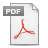Verma, S. P., Díaz-González, L., Pérez-Garza, J. A., Rosales-Rivera, M. (2016). Quality control in geochemistry from a comparison of four central tendency and five dispersion estimators and example of a geochemical reference material. Arabian Journal of Geosciences, 9 (20), 740.

 Quality control in geochemistry from a comparison of four central tendency and five dispersion estimators and example of a geochemical reference material Data quality control in geochemistry constitutes a fundamental problem that is still to be solved from the application of statistics and computation. We used refined Monte Carlo simulations of 10,000 replications and 190 independent experiments for sample sizes of 5 to 100. Statistical contaminations of 1 to 4 observations were used to compare 9 statistical parameters (4 central tendency—mean, median, trimean, and Gastwirth mean, and 5 dispersion estimates— standard deviation, median absolute deviation, Sn, Qn, and σn ). The presence of discordant observations in the data arrays rendered the outlier-based and robust parameters to disagree with each other. However, when the mean and standard deviation (outlier-based parameters) were estimated from censored data arrays obtained after the identification and separation of outlying observations, they generally provided a better estimate of the population than the robust estimates obtained from the original data arrays. This inference is contrary to the general belief, and therefore, reasons for the better performance of the outlier-based methods as compared to the robust methods are suggested. However, when all parameters were estimated from censored arrays and appropriate precise and accurate correction factors put forth in this work were applied, all of them became fully consistent, i.e., the mean agreed with the median, trimean and Gastwirth mean, and the standard deviation with the median absolute deviation, Sn, Qn, and σn. An example of inter-laboratory chemical data for a Hawaiian reference material BHVO-1 included sample sizes from 5 to 100, which showed that small samples of up to 20 provide inconsistent estimates, whereas larger samples of 20–100, especially >40, were more appropriate for estimating statistical parameters through robust or outlier-based methods. Although all statistical estimators provided consistent results, our simulation study shows that it is better to use the censored sample mean and population standard deviation as the best estimates